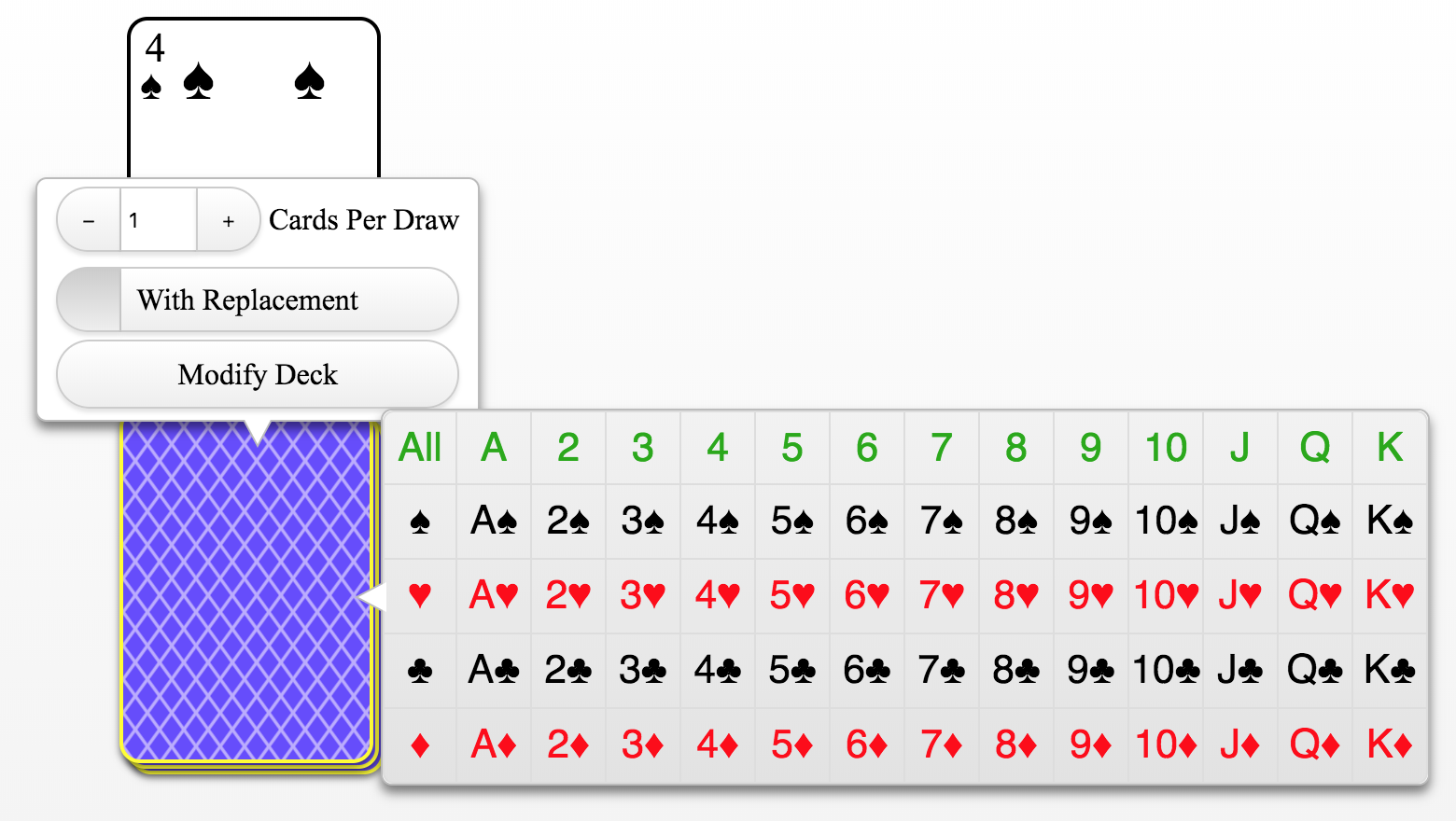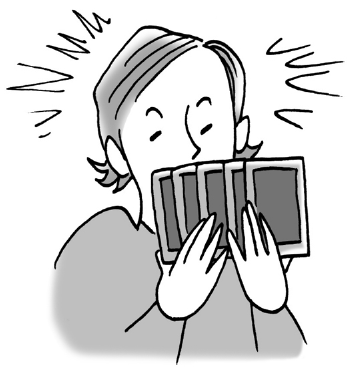### Home > INT2 > Chapter 3 > Lesson 3.1.4 > Problem3-41

3-41.

Avery has been learning to play some new card games and is curious about the probabilities of being dealt different cards from a standard $52$-card deck. Help him figure out the probabilities listed below. .

1. What are P(king), P(queen), and P(club)?

How many kings are in a deck of cards? Queens? Clubs?2. What is P(king or club)? How does your answer relate to the probabilities you calculated in part (a)?

Use the Addition Rule to eliminate P(king and club).

$P \text{(king or club)}= \frac{16}{52}$

3. What is P(king or queen)? Again, how does your answer relate to the probabilities you calculated in part (a)?